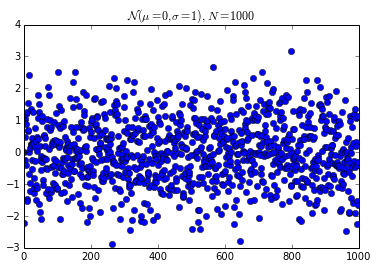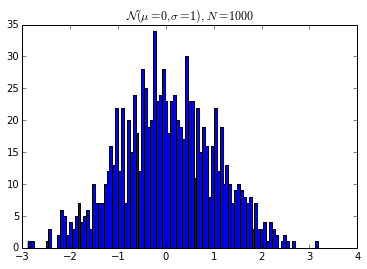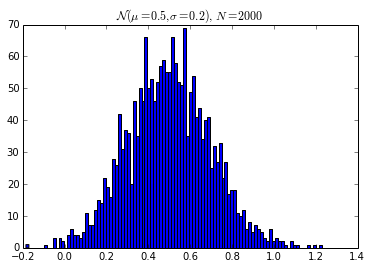# Defining Custom Display Logic for Your Own Objects¶

## Overview¶

In Python, objects can declare their textual representation using the __repr__ method. IPython expands on this idea and allows objects to declare other, richer representations including:

• HTML
• JSON
• PNG
• JPEG
• SVG
• LaTeX

This Notebook shows how you can add custom display logic to your own classes, so that they can be displayed using these rich representations. There are two ways of accomplishing this:

1. Implementing special display methods such as _repr_html_.
2. Registering a display function for a particular type.

In this Notebook we show how both approaches work.

Parts of this notebook need the inline matplotlib backend:

In :
%matplotlib inline
import numpy as np
import matplotlib.pyplot as plt


## Implementing special display methods¶

The main idea of the first approach is that you have to implement special display methods, one for each representation you want to use. The names of the special methods are self explanatory:

• _repr_html_
• _repr_json_
• _repr_jpeg_
• _repr_png_
• _repr_svg_
• _repr_latex_

As an illustration, we build a class that holds data generated by sampling a Gaussian distribution with given mean and variance. Each frontend can then decide which representation it will display be default. Further, we show how to display a particular representation.

The next cell defines the Gaussian class:

In :
from IPython.core.pylabtools import print_figure
from IPython.display import Image, SVG, Math

class Gaussian(object):
"""A simple object holding data sampled from a Gaussian distribution.
"""
def __init__(self, mean=0, std=1, size=1000):
self.data = np.random.normal(mean, std, size)
self.mean = mean
self.std = std
self.size = size
# For caching plots that may be expensive to compute
self._png_data = None
self._svg_data = None

def _figure_data(self, format):
fig, ax = plt.subplots()
ax.plot(self.data, 'o')
ax.set_title(self._repr_latex_())
data = print_figure(fig, format)
# We MUST close the figure, otherwise IPython's display machinery
# will pick it up and send it as output, resulting in a double display
plt.close(fig)
return data

# Here we define the special repr methods that provide the IPython display protocol
# Note that for the two figures, we cache the figure data once computed.

def _repr_png_(self):
if self._png_data is None:
self._png_data = self._figure_data('png')
return self._png_data

def _repr_svg_(self):
if self._svg_data is None:
self._svg_data = self._figure_data('svg').decode('utf-8')#.encode('utf-8')
return self._svg_data

def _repr_latex_(self):
return r'$\mathcal{N}(\mu=%.2g, \sigma=%.2g),\ N=%d$' % (self.mean,
self.std, self.size)

# We expose as properties some of the above reprs, so that the user can see them
# directly (since otherwise the client dictates which one it shows by default)
@property
def png(self):
return Image(self._repr_png_(), embed=True)

@property
def svg(self):
return SVG(self._repr_svg_())

@property
def latex(self):
return Math(self._repr_latex_())

# An example of using a property to display rich information, in this case
# the histogram of the distribution.  We've hardcoded the format to be png
# in this case, but in production code it would be trivial to make it an option
@property
def hist(self):
fig, ax = plt.subplots()
ax.hist(self.data, bins=100)
ax.set_title(self._repr_latex_())
data = print_figure(fig, 'png')
plt.close(fig)
return Image(data, embed=True)


Now, we create an instance of the Gaussian distribution, whose default representation will be its LaTeX form:

In :
x = Gaussian()
x

Out:

We can view the data in png or svg formats:

In :
x.png

Out:In :
x.svg

Out:

Since IPython only displays by default as an Out[] cell the result of the last computation, we can use the display() function to show more than one representation in a single cell:

In :
from IPython.display import display

In :
display(x.png)
display(x.svg)Now let's create a new Gaussian with different parameters

In :
x2 = Gaussian(0.5, 0.2, 2000)
x2

Out:

We can easily compare them by displaying their histograms

In :
display(x.hist)
display(x2.hist)## Adding IPython display support to existing objects¶

When you are directly writing your own classes, you can adapt them for display in IPython by following the above example. But in practice, we often need to work with existing code we can't modify. We now illustrate how to add these kinds of extended display capabilities to existing objects. We will use the NumPy polynomials and change their default representation to be a formatted LaTeX expression.

First, consider how a numpy polynomial object renders by default:

In :
p = np.polynomial.Polynomial([1,2,3], [-10, 10])
p

Out:
Polynomial([ 1.,  2.,  3.], [-10.,  10.], [-1.,  1.])

Next, we define a function that pretty-prints a polynomial as a LaTeX string:

In :
def poly2latex(p):
terms = ['%.2g' % p.coef]
if len(p) > 1:
term = 'x'
c = p.coef
if c!=1:
term = ('%.2g ' % c) + term
terms.append(term)
if len(p) > 2:
for i in range(2, len(p)):
term = 'x^%d' % i
c = p.coef[i]
if c!=1:
term = ('%.2g ' % c) + term
terms.append(term)
px = '$P(x)=%s$' % '+'.join(terms)
dom = r', domain: $[%.2g,\ %.2g]$' % tuple(p.domain)
return px+dom


This produces, on our polynomial p, the following:

In :
poly2latex(p)

Out:
'$P(x)=1+2 x+3 x^2$, domain: $[-10,\\ 10]$'
In :
from IPython.display import Latex
Latex(poly2latex(p))

Out:
$P(x)=1+2 x+3 x^2$, domain: $[-10,\ 10]$

But we can configure IPython to do this automatically for us as follows. We hook into the IPython display system and instruct it to use poly2latex for the latex mimetype, when encountering objects of the Polynomial type defined in the numpy.polynomial.polynomial module:

In :
ip = get_ipython()
latex_formatter = ip.display_formatter.formatters['text/latex']
latex_formatter.for_type_by_name('numpy.polynomial.polynomial',
'Polynomial', poly2latex)


For more examples on how to use the above system, and how to bundle similar print functions into a convenient IPython extension, see sympy's printing extension.

Once our special printer has been loaded, all polynomials will be represented by their mathematical form instead:

In :
p

Out:
$P(x)=1+2 x+3 x^2$, domain: $[-10,\ 10]$
In :
p2 = np.polynomial.Polynomial([-20, 71, -15, 1])
p2

Out:
$P(x)=-20+71 x+-15 x^2+x^3$, domain: $[-1,\ 1]$

## More complex display with _ipython_display_¶

Rich reprs can only display one object or mime-type at a time. Sometimes this is not enough, because you need to get javascript on the page, or you want LaTeX and a PNG. This can be done with multiple calls to display easily enough, but then users need to know more than they should.

In IPython 2.0, we added a special _ipython_display_ method, that allows your objects to take control of displaying. If this method is defined, IPython will call it, and make no effort to display the object itself. It's a way for you to say "Back off, IPython, I got this."

In :
import json
import uuid
from IPython.display import display_javascript, display_html, display

class FlotPlot(object):
def __init__(self, x, y):
self.x = x
self.y = y
self.uuid = str(uuid.uuid4())

def _ipython_display_(self):
json_data = json.dumps(zip(self.x, self.y))
display_html('<div id="{}" style="height: 300px; width:80%;"></div>'.format(self.uuid),
raw=True
)
display_javascript("""
require(["//cdnjs.cloudflare.com/ajax/libs/flot/0.8.2/jquery.flot.min.js"], function() {
var line = JSON.parse("%s");
console.log(line);
\$.plot("#%s", [line]);
});
""" % (json_data, self.uuid), raw=True)

In :
import numpy as np
x = np.linspace(0,10)
y = np.sin(x)
FlotPlot(x, np.sin(x))